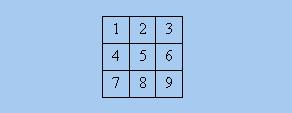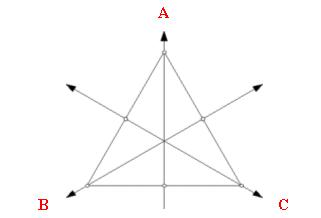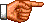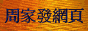# 點算的奧秘：著色方案的母函數

《點算的奧秘：伯恩賽德-波利亞定理》中，筆者介紹了求解「著色」問題的「伯恩賽德-波利亞定理」。上節的「著色」問題都是一般的「著色」問題，這些「著色」問題除了對「物件」(即幾何圖形的「部件」)集合O、「顏色」集合C和「運動」集合G有所規定外，對所求的「著色」方案別無其他規定。可是，在某些應用中，我們所求的答案不是全體「著色」方案的數目，而是受限制的「著色」方案的數目。本文主旨就是介紹這些「受限制的著色問題」的求解方法。我們以一個例題開始。

D4的元素g「循環式」「循環類型」Z[g*]「母函數」 |Fix(g)|
e(1)(2)(3)(4) z14(o + g)4 24 = 16
r(1 2 3 4)z4 o4 + g42
r2(1 3)(2 4) z22 (o2 + g2)222 = 4
r3(1 4 3 2) z4o4 + g4 2
v(1 2)(3 4) z22 (o2 + g2)222 = 4
h(1 4)(2 3) z22 (o2 + g2)222 = 4
m(1 3)(2)(4) z12z2 (o + g)2(o2 + g2) 23 = 8
n(1)(2 4)(3) z12z2 (o + g)2(o2 + g2) 23 = 8

(o + g)4 = o4 + 4o3g + 6o2g2 + 4og3 + g4

 [(o + g)4 + 2(o4 + g4) + 3(o2 + g2)2 + 2(o + g)2(o2 + g2)] / 8 = o4 + o3g + 2o2g2 + og3 + g4    (1)

 Z[D4](z1, z2, z4) = Σg ∈ D4 Z[g*] / |D4| = (z14 + 2z4 + 3z22 + 2z12z2) / 8

Z[D4](o + g, o2 + g2, o4 + g4)

|F / G| = Z[G](c, c, ... c)

Z[G](C1 + C2 + ... Cc, C12 + C22 + ... Cc2, ... C1n + C2n + ... Ccn)Z[D4](o + g + w, o2 + g2 + w2, o4 + g4 + w4) = [(o + g + w)9 + 2(o + g + w)(o4 + g4 + w4)2 + (o + g + w)(o2 + g2 + w2)4 + 4(o + g + w)3(o2 + g2 + w2)3] / 8

9! / (4! 3! 2!) = 1260

4! / (2! 1! 1!) = 12

4 × 3! / (2! 0! 1!) = 12

4 × [3! / (1! 2!)] × [3! / (2! 1!)] = 36

4 × [3! / (1! 2!)] × [3! / (1! 1! 1!)] = 72

(1260 + 12 + 12 + 36 + 72) / 8 = 174 □D3的元素gg*的「循環式」Z[g*]
e(A)(B)(C)z13
r(A B C)z3
r2(A C B)z3
a(A)(B C)z1z2
b(A C)(B)z1z2
c(A B)(C)z1z2

Z[D3](z1, z2, z3) = (z13 + 3z1z2 + 2z3) / 6

Z[D3](3, 3, 3) = (33 + 3 × 3 × 3 + 2 × 3) / 6 = 10

 Z[D3](o + g + w, o2 + g2 + w2, o3 + g3 + w3) = [(o + g + w)3 + 3(o + g + w)(o2 + g2 + w2) + 2(o3 + g3 + w3)] / 6 = o3 + g3 + w3 + o2g + og2 + o2w + ow2 + g2w + gw2 + ogw